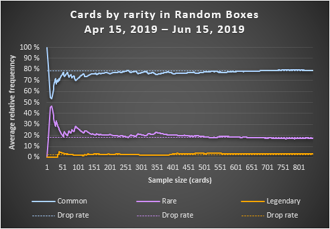## FANDOM

5,647 Pages

In statistics, the term frequency is used to describe the occurrence of events, not in a theoretical sample space but in an actual experiment.

## Absolute frequency

The frequency (or absolute frequency) of an event is the number of times the event occurred in an experiment or study. As an absolute frequency is the result of counting, it is always a natural number and cannot be negative.

Example: If 2 are opened and grant 7 rare cards, then the absolute frequency of rare cards is 7 (more precisely, the event of getting a rare card occured with an absolute frequency of 7).

## Relative frequency

The relative frequency (or empirical probability) of an event is the absolute frequency divided by the total number of events. It is a fraction and has a value between 0 and 1.

Example: As a Daily Kit Box contains 10 cards, opening 2 of them will result in a total number of 20 cards. If 7 of them were rare, then the event of getting a rare card occured 7 out of 20 times. In other words: The relative frequency of getting a rare card is 7/20 = 0.35 or 35 %.

Relative frequencies are useful to make experiments comparable, regardless of the sample size. Furthermore, they can be used to obtain information about probabilities: As it follows from the law of large numbers that the average relative frequency of an event will converge to its expected value and, under certain conditions, to its probability, opening a large number of Pro Kit Boxes can help to

• verify drop rates provided in box infos and
• infer probabilities of getting certain cards from a box.

## Relative frequency and drop rate

Drop rates in box infos are provided in percent and denote the average relative frequency of a card in the long run, when a large number of boxes is opened. This means that the content of one single box can deviate significantly from the drop rates, but the more boxes are opened, the closer the average amount of cards of each rarity will be to the official drop rates. In other words:

Drop rates are expected values of average relative frequencies.Example: The line chart to the right shows the average relative frequency of common, rare and legendary cards obtained from . In this case, the first box was a Random Box 1 that contained a common card, so 100 % of all drawn cards were common. After that, an unusually high (but possible) amount of rare cards appeared which resulted in around 45 % of all cards being rare. A player not familiar with the concept of drop rates and expected values could think that the box info was plain wrong. It took more than 150 cards for the average values to finally approach the official drop rates marked by the broken lines.